Courses

# RD Sharma Solutions - Ex - 7.1, Algebraic Expressions, Class 7, Math Class 7 Notes | EduRev

## RD Sharma Solutions for Class 7 Mathematics

Created by: Abhishek Kapoor

## Class 7 : RD Sharma Solutions - Ex - 7.1, Algebraic Expressions, Class 7, Math Class 7 Notes | EduRev

The document RD Sharma Solutions - Ex - 7.1, Algebraic Expressions, Class 7, Math Class 7 Notes | EduRev is a part of the Class 7 Course RD Sharma Solutions for Class 7 Mathematics.
All you need of Class 7 at this link: Class 7

#### Question 1:

Identify the monomials, binomials, trinomials and quadrinomials from the following expressions:
(i) a2
(ii) a2 − b2
(iii) x3y3z3
(iv) x3y3z3 + 3xyz
(v) 7 + 5
(vi) abc + 1
(vii) 3x − 2 + 5
(viii) 2x − 3x + 4
(ix) xyyzzx
(x) ax3bc2cxd

The monomials, binomials, trinomials and quadrinomials are as follows.
(i) a2 is a monomial expression as it contains one term only.
(ii) a2 - b2 is a binomial expression as it contains two terms.
(iii) x3 + y3 + z3 is a trinomial expression as it contains three terms.
(iv) x3 + y3 + z3 + xyz is a quadrinomial expression as it contains four terms.
(v) 7 + 5 = 12 is a monomial expression as it contains one term only.
(vi) abc +1 is a binomial expression as it contains two terms.
(vii) 3x - 2 + 5 = 3x + 3 is a binomial expression as it contains two terms.
(viii) 2x - 3y + 4 is a trinomial expression as it contains three terms.
(ix) xy + yz + zx is a trinomial expression as it contains three terms.
(x) ax3 +bx2 +cx + d is a quadrinomial expression as it contains four terms.

#### Question 2:

Write all the terms of each of the following algebraic expressions:
(i) 3x
(ii) 2x − 3
(iii) 2x2 − 7
(iv) 2x2y2 − 3xy + 4

The terms of each of the given algebraic expressions are as follows.
(i) 3x is the only term of the given algebraic expression.
(ii) 2x and -3 are the terms of the given algebraic expression.
(iii) 2x2 and -7 are the terms of the given algebraic expression.
(iv) 2x2, y2, -3xy  and 4 are the terms of the given algebraic expression.

#### Question 3:

Identify the like terms and also mention the numerical coefficients of those terms:

(i) 4xy, −5x2y, −3yx, 2xy2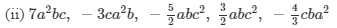like terms Numerical Cofficients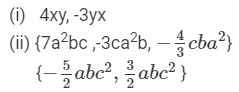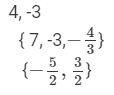#### Question 4:

Identify the like terms in the following algebraic expressions:

(i) a2+b2−2a2+c2+4a

(ii) 3x+4xy−2yz+5/2zy
(iii) abc+ab2c+2acb2+3c2ab+b2ac−2a2bc+3cab2

The like terms in the given algebraic expressions are as follows.
(i) The like terms in the given algebraic expression are a2  and -2a2.

(ii) The like terms in the given algebraic expression are −2yz and 52zy-2yz and 5/2zy.
(iii) The like terms in the given algebraic expression are ab2c, 2acb2, b2ac and 3cab2.

#### Question 5:

Write the coefficient of x in the following:
(i) −12x
(ii) −7xy
(iii) xyz
(iv) −7

The coefficients of x are as follows.
(i) The numerical coefficient of x is -12.
(ii) The numerical coefficient of x is -7y.
(iii) The numerical coefficient of x is yz.
(iv) The numerical coefficient of x is -7a.

#### Question 6:

Write the coefficient of x2 in the following:
(i) −3x2
(ii) 5x2yx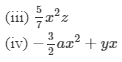The coefficients of x2 are as follows.
(i) The numerical coefficient of x2 is -3.
(ii) The numerical coefficient of x2 is 5yz.

(iii) The numerical coefficient of x2 is 5/7z.
(iv) The numerical coefficient of x2 is −3/2a.

#### Question 7:

Write the coefficient of:
(i) y in −3y
(ii) a in 2ab
(iii) z in −7xyz
(iv) p in −3pqr
(v) y2 in 9xy2z
(vi) x3 in x3 + 1
(vii) x2 in −x2

The coefficients are as follows.

(i) The coefficient of  y is -3.
(ii) The coefficient of  a is 2b.
(iii) The coefficient of  z is -7xy.
(iv) The coefficient of  p is -3qr.
(v) The coefficient of  y2 is 9xz.
(vi) The coefficient of  x3 is 1.
(vii) The coefficient of  -x2 is - 1.

#### Question 8:

Write the numerical coefficient of each of the following:
(i) xy
(ii) −6yz
(iii) 7abc
(iv) −2x3y2z

The numerical coefficient of each of the given terms is as follows.
(i) The numerical coefficient in the term xy is 1.
(ii) The numerical coefficient in the term -6yz is - 6.
(iii) The numerical coefficient in the term 7abc is 7.
(iv) The numerical coefficient in the term -2x3y2z is - 2.

#### Question 9:

Write the numerical coefficient of each term in the following algebraic expressions: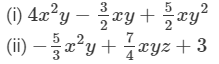The numerical coefficient of each term in the given algebraic expressions is as follows.

 Term Coefficient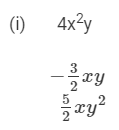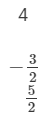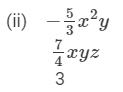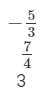Question 10:

Write the constant term of each of the following algebraic expressions:
(i) x2y − xy2 + 7xy − 3
(ii) a3 − 3a2 + 7a + 5

The constant term of each of the given algebraic expressions is as follows.
(i) The constant term in the given algebraic expression is -3.
(ii) The constant term in the given algebraic expression is 5.

#### Question 11:

Evaluate each of the following expressions for x = − 2, y = −1, z = 3: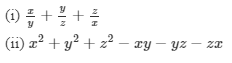We have x = −2, y = −1 and z = 3.
Thus,
(i)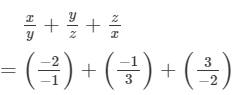(ii) x2 + y2 + z2- xy - yz - zx
= (-2)2 + (-1)2 + (3)2 - (-2)(-1) - (-1)(3) - (3)(-2)
= 4 + 1 + 9 - 2 + 3 + 6
= (4 + 1 + 9 + 3 + 6) - 2
= 23 - 2 = 21

#### Question 12:

Evaluate each of the following algebraic expressions for x = 1, y = −1, z = 2, a = −2, b = 1, c = −2:

(i) axbycz
(ii) ax2by2 − cz2
(iii) axybyz + cxy

We have x = 1, y = −1, z = 2, a = −2, b = 1 and c = −2.
Thus,
(i) ax + by + cz
= (-2)(1) + (1)(-1) + (-2)(2)
= - 2 + (- 1) + (- 4)
= - 2 -1 - 4 = -7

(ii) ax2 + by2 - cz2
= (-2)(1)2 + (1)(-1)2 - (-2)(2)2
= -2 x 1 + 1 - (-2 x 4)
= -2 + 1 - (-8)
= -2 + 1 + 8
= -2 + 9
= 7

(iii) axy + byz + cxy
= (-2)(1)(-1) + (1)(-1)(2) + (-2)(1)(-1)
= 2 + (-2) + 2
= 2 - 2 + 2
= 4 - 2
= 2

97 docs

,

,

,

,

,

,

,

,

,

,

,

,

,

,

,

,

,

,

,

,

,

,

,

,

,

,

,

,

,

,

;